Updating search results...# NSDL Math Common Core

The NSDL Math Common Core collection provides quick and easy access to high-quality math resources that have been related to one or more standard statements within the Math Common Core. These resources are selected from the larger NSDL collection and other trusted providers, and organized by grade level and domain area.

# 41 Results

View
Selected filters:
• NSDL Math Common CoreRating
0.0 stars

This online manipulative features a virtual balance scale. It offers students an experimental way to learn about solving linear equations involving negative or positive numbers. The applet presents an equation for the student to illustrate by balancing the scale using blue blocks for positive units and variables and red balloons for negative units and variables. The student then works with the arithmetic operations to solve the equation. A record of the steps taken by the student is shown on the screen and on the scale. The applet reinforces the idea that what is done to one side of an equation must be done to the other side to maintain balance. Instructions for using the applet, background information about solving equations, and teaching suggestions are included.

Subject:
Mathematics
Algebra
Material Type:
Activity/Lab
Interactive
Provider:
NSDL Staff
Provider Set:
NSDL Math Common Core
03/31/2003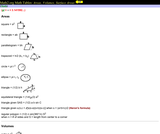Rating
0.0 stars

This printable sheet is an excellent reference tool for geometry students. It details the formulae for finding the area, volume, and surface area for a variety of two- and three-dimensional shapes and includes an illustration of each that shows which measurements are important to the calculation. Presented are: areas of polygons (square, rectangle, parallelogram, trapezoid, circle, ellipse, triangles); volumes of polyhedra (cube, rectangular prism, irregular prism, cylinder, pyramid, cone, sphere, ellipsoid); and surface area (cube, prism, sphere).

Subject:
Mathematics
Material Type:
Student Guide
Provider:
NSDL Staff
Provider Set:
NSDL Math Common Core
Author:
David Manura
10/18/2002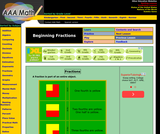Rating
0.0 stars

For the learner just being introduced to fractions, this site is an excellent resource. It contain a very basic explanation of what a fraction is and a game for students to practice naming the fraction presented. The game can also be manipulated to have a time limit, to award time for each correct answer, and to time how fast students can get 20 more answers right than wrong.

Subject:
Mathematics
Material Type:
Activity/Lab
Game
Provider:
NSDL Staff
Provider Set:
NSDL Math Common Core
Author:
J.C. Banfill
12/12/2007Rating
0.0 stars

This ready-to-print work sheet presents students with three problems, and in order to solve them, students must convert between smaller and larger units and use all four mathematical operations. There are a total of seven questions involving these three problems, and the conversions which students must undertake involve dollars and cents, pounds and ounces, grams and kilograms, and centimeters and meters.

Subject:
Mathematics
Material Type:
Lecture Notes
Provider:
NSDL Staff
Provider Set:
NSDL Math Common Core
Author:
Nancy Molik
03/31/2004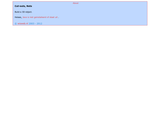Rating
0.0 stars

This interactive applet allows students to explore cut-outs of polyhedra, including a tetrahedron, cube, octahedron, dodecahedron, and icosahedron. The applet begins with each shape "unfolded" to its two-dimensional form. The slider bar allows students to fold the shape into its three-dimensional form. Students can experiment by folding the sides independently and rotating the shape. This applet can help students understand concepts of surface area and decomposing 3D shapes into 2D elements.

Subject:
Mathematics
Material Type:
Interactive
Lecture Notes
Provider:
NSDL Staff
Provider Set:
NSDL Math Common Core
Author:
Peter Boon
03/31/2004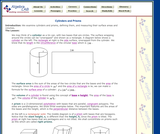Rating
0.0 stars

In this lesson, students will examine cylinders and prisms, define them, and measure their surface areas and volumes. The site has clear illustrations, translating the shapes to 2-dimensional figures, and practice problems for educators to solve with the students. There are two example problems at the end that students may tackle alone to solidify their understanding.

Subject:
Mathematics
Algebra
Material Type:
Lecture Notes
Student Guide
Provider:
NSDL Staff
Provider Set:
NSDL Math Common Core
Author:
Marshall Ransom
03/31/2005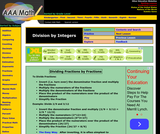Rating
0.0 stars

This site gives a description of how to divide fractions and gives a sample problem in which the denominator fraction is inverted, the numerators and denominators are multiplied, and the resulting fraction is simplified. It also includes the same problem solved by 'canceling' the fractions to simplify, and completing the process. There is also a game for students to practice their new skill of dividing and simplifying fractions.

Subject:
Mathematics
Material Type:
Activity/Lab
Game
Provider:
NSDL Staff
Provider Set:
NSDL Math Common Core
Author:
J.C. Banfill
12/12/2007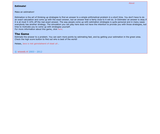Rating
0.0 stars

This interactive game asks students to estimate an answer to various problems. While the site does not teach students individual strategies for estimating, it allows them to practice and come up with their own. Answers are considered correct when they are within 10% of the actual answer.

Subject:
Mathematics
Material Type:
Interactive
Lecture Notes
Simulation
Provider:
NSDL Staff
Provider Set:
NSDL Math Common Core
Author:
Michiel Doorman
03/31/2004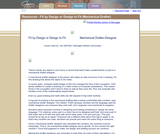Rating
0.0 stars

This applied mathematics lesson features hands-on activities supporting the NCTM Measurement Standard, and can be used to introduce middle school students to a bit of real-world measurement, trigonometry, and geometry through mechanical drafting. All that is needed are measurement tools ŰÓ carpenterŰŞs square, a piece of string, and a protractor. In it, students read an introduction about the importance and various uses of applied trigonometry and practice by examining the classroom and identifying which objects would have been designed by a mechanical drafter. They then discuss which aspects of the object would need to be measured and how those measurements would influence the design. Students practice by placing a string across the carpenter's square and use the Pythagorean Theorem to perform calculations on the right triangles produced. There are a series of extra activities in which students measure classroom objects to solve practical problems.

Subject:
Mathematics
Material Type:
Activity/Lab
Lesson Plan
Provider:
NSDL Staff
Provider Set:
NSDL Math Common Core
Author:
Van McPhail
03/31/2008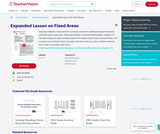Some Rights Reserved
Rating
0.0 stars

In this activity, students explore area and perimeter, the difference between them, and how to calculate them. As an introductory activity, students are given 12 tiles and asked to design a rectangle from them and uncover the definitions of area and perimeter. Then, students are given 36 tiles and asked to create as many rectangles as possible, observing the dimensions, area, and perimeter of each. A worksheet is provided, along with centimeter and half-centimeter grid paper.

Subject:
Mathematics
Material Type:
Activity/Lab
Lecture Notes
Lesson Plan
Provider:
NSDL Staff
Provider Set:
NSDL Math Common Core
Author:
John Van de Walle
Lou Ann H. Lovin
03/31/2006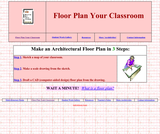Rating
0.0 stars

This resource guides the learner, step-by-step, in creating a scale floor plan of a classroom. The instructions include sample drawings of student work. The activity includes: sketching a map of the classroom; measuring the room and calculating the area and perimeter; creating a scale drawing; and drafting a CAD (computer-aided design) floor plan. The lesson provides students with hands-on, real world practice solving problems of measurement, ratio, and scale.

Subject:
Mathematics
Material Type:
Activity/Lab
Lesson Plan
Provider:
NSDL Staff
Provider Set:
NSDL Math Common Core
03/31/2003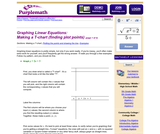Some Rights Reserved
Rating
0.0 stars

From Elizabeth Stapel at Purplemath, this module helps students understand how to graph linear equations by making a neat T-chart, finding plot points, plotting points, and drawing the line. There are four pages in this module with clear, systematically presented, step-by-step instructions and plenty of examples and illustrations to help students along.

Subject:
Mathematics
Material Type:
Lesson Plan
Unit of Study
Provider:
NSDL Staff
Provider Set:
NSDL Math Common Core
Author:
Elizabeth Stapel
04/14/2000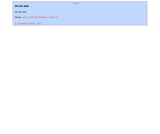Rating
0.0 stars

This game presents students with a number and asks them to find all the factors (divisors) of it between 1-10. There are two modes: the first uses numbers 1-100 and the second uses 101-999. Students then select which numbers are factors and try to open the safe. If they are correct, the safe will open. If they are incorrect, the simulation gives a hint as to how many they have correct and two more tries. There is a calculator built in to the site for students to use to help solve each problem.

Subject:
Mathematics
Material Type:
Interactive
Lecture Notes
Simulation
Provider:
NSDL Staff
Provider Set:
NSDL Math Common Core
Author:
Frans Moerlands
03/31/2004Rating
0.0 stars

This applet allows students to freely build shapes by stacking cubes and "explore the relation between a building (house) consisting of cubes and the height numbers representing the height of the different parts of the building." This exercise helps students visualize and understand the concepts of volume and three-dimensional, measurable space.

Subject:
Mathematics
Material Type:
Interactive
Simulation
Provider:
NSDL Staff
Provider Set:
NSDL Math Common Core
Author:
Jan van den Brink
Peter Boon
03/31/2004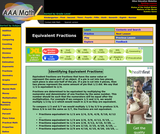Rating
0.0 stars

This site helps students understand how to identify equivalent fractions by explaining what equivalent fractions are and how to compare two fractions to see if they are equivalent. There is also a list of fractions equivalent to 1/2, 1/3, 1/4, 1/5, and 2/5 so students can begin to see the pattern that emerges. Finally, the site presents an interactive game in which students can practice by naming the equivalent fraction to a given fraction.

Subject:
Mathematics
Material Type:
Activity/Lab
Game
Provider:
NSDL Staff
Provider Set:
NSDL Math Common Core
Author:
J.C. Banfill
12/12/2007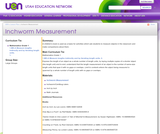Some Rights Reserved
Rating
0.0 stars

In this activity, students are introduced to the concept of measuring length. Students estimate and then use inchworm measuring tools to measure the lengths of 10 classroom objects, and record their estimations and measurements on a worksheet. As an introduction, students may also read "Inch by Inch" by Leo Lionni. This lesson includes a printable student worksheet in PDF format.

Subject:
Mathematics
Material Type:
Activity/Lab
Lecture Notes
Lesson Plan
Provider:
NSDL Staff
Provider Set:
NSDL Math Common Core
Author:
Holly Barron
01/30/2001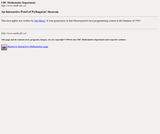Rating
0.0 stars

This interactive simulation, students are able to visualize a proof of the Pythagorean Theorem by choosing a right triangle, and watching as a2 + b2 = c2 is proved. This is an excellent introduction for students to not only understand the formula, but why the formula works to measure the sides of right triangles.

Subject:
Mathematics
Material Type:
Interactive
Provider:
NSDL Staff
Provider Set:
NSDL Math Common Core
Author:
Jim Morey
03/31/1995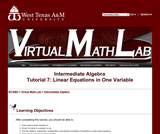Some Rights Reserved
Rating
0.0 stars

This lesson introduces students to linear equations in one variable; shows how to solve them using addition, subtraction, multiplication, and division properties of equalities; and allows them to determine if a value is a solution, if there are infinitely many solutions, or no solution at all. The site contains an explanation of equations and linear equations, how to solve equations in general, and a strategy for solving linear equations. The lesson also explains contradiction (an equation with no solution) and identity (an equation with infinite solutions). There are five practice problems at the end for students to test their knowledge with links to answers and explanations of how those answers were found.

Subject:
Mathematics
Algebra
Material Type:
Lecture Notes
Provider:
NSDL Staff
Provider Set:
NSDL Math Common Core
Author:
Jennifer Puckett
Kim Seward
03/31/2008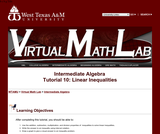Some Rights Reserved
Rating
0.0 stars

Upon completing this lesson, student should be able to use the addition, subtraction, multiplication, and division properties of inequalities to solve linear inequalities; write the answer to an inequality using interval notation; and draw a graph to give a visual answer to an inequality problem. The lesson begins with explanations of inequality signs and interval notation and then moves on to demonstrate addition/subtraction and multiplication/division properties in inequalities. The site demonstrates a strategy for solving linear inequalities and presents three problems for students to practice what they have learned. There is also a link to a previous tutorial which covers solving linear equations of one variable for students who need the review.

Subject:
Mathematics
Algebra
Material Type:
Lecture Notes
Provider:
NSDL Staff
Provider Set:
NSDL Math Common Core
Author:
Jennifer Puckett
Kim Seward
03/31/2008Rating
0.0 stars

This lesson helps students understand and practice converting measurements in both English and metric systems. The 8-page pdf begins with a brief introduction to measuring length, English measurement systems, and metric measurement. Students then learn to convert measurements within each system. At the end of the lesson, there are 18 review problems for students to convert measurements on their own. Answers are provided.

Subject:
Mathematics
Material Type:
Lecture Notes
Lesson Plan
Student Guide
Provider:
NSDL Staff
Provider Set:
NSDL Math Common Core